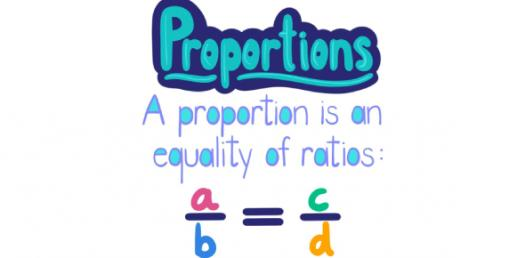# 7th Grade Math Proportion Word Problems!

Approved & Edited by ProProfs Editorial Team
The editorial team at ProProfs Quizzes consists of a select group of subject experts, trivia writers, and quiz masters who have authored over 10,000 quizzes taken by more than 100 million users. This team includes our in-house seasoned quiz moderators and subject matter experts. Our editorial experts, spread across the world, are rigorously trained using our comprehensive guidelines to ensure that you receive the highest quality quizzes.
| By Fletcher Bowen
F
Fletcher Bowen
Community Contributor
Quizzes Created: 2 | Total Attempts: 33,532
Questions: 10 | Attempts: 680SettingsAre you any good with mathematical word problems? Give this quiz a try. A word problem in mathematics education is generally used to refer to any mathematical exercise in which an example consisting of words and numbers is given. The problem is given more in words, as opposed to mathematical notation. Taking this quiz will help you know about mathematical word problems.

• 1.

### Tim can buy 8 apples for 2 dollars, how much would it cost Tim to buy 25 apples?

Explanation
If Tim can buy 8 apples for 2 dollars, it means that each apple costs 2/8 = 0.25 dollars. To find out how much it would cost Tim to buy 25 apples, we can multiply the cost of one apple (0.25 dollars) by the number of apples (25), which gives us 0.25 * 25 = 6.25 dollars. Therefore, it would cost Tim 6.25 dollars to buy 25 apples.

Rate this question:

• 2.

### Larry eats 5 Skittles every minute, how many Skittles can Larry eat in 30 minutes?

Explanation
Larry eats 5 Skittles every minute, and we want to find out how many Skittles he can eat in 30 minutes. To do this, we can multiply the number of Skittles he eats per minute (5) by the number of minutes (30). This gives us 5 x 30 = 150. Therefore, Larry can eat 150 Skittles in 30 minutes.

Rate this question:

• 3.

### Tara can run 4 laps in 11 minutes, how long would it take Tara to run 1 lap?

Explanation
Tara can run 4 laps in 11 minutes, which means she runs 1 lap in 11/4 minutes. To find out how long it would take Tara to run 1 lap, we divide 11 by 4, which equals 2.75. Therefore, it would take Tara 2.75 minutes to run 1 lap.

Rate this question:

• 4.

### Stacey can buy 10 pies for \$30, how much would it cost Stacey to buy 2 pies?

Explanation
If Stacey can buy 10 pies for \$30, then the cost per pie would be \$3. Therefore, if Stacey wants to buy 2 pies, it would cost her 2 multiplied by \$3, which equals \$6.

Rate this question:

• 5.

### Michael can play one hole of golf in 13 minutes, how long would it take Michael to finish one full round of golf (18 holes)?

Explanation
If Michael can play one hole of golf in 13 minutes, then it would take him the same amount of time to play each of the 18 holes. Therefore, it would take Michael 234 minutes to finish one full round of golf (18 holes).

Rate this question:

• 6.

### Andrea walks 5 miles per hour, how many miles can Andrea walk in 4 hours

Explanation
Andrea walks at a speed of 5 miles per hour. Therefore, in 1 hour, she can walk 5 miles. If we multiply this by 4 hours, we can calculate that Andrea can walk a total of 20 miles in 4 hours.

Rate this question:

• 7.

### Veronica bought 2 pairs of jeans for \$23, how much would 3 pairs of jeans cost?

Explanation
If Veronica bought 2 pairs of jeans for \$23, then the cost of each pair of jeans would be \$23 divided by 2, which is \$11.50. Therefore, if she wants to buy 3 pairs of jeans, the total cost would be \$11.50 multiplied by 3, which equals \$34.50.

Rate this question:

• 8.

### Dustin can eat 4 hotdogs in one minute, if he enters a hotdog eating contest that last 15 minutes, how many hotdogs would he consume?

Explanation
Dustin can eat 4 hotdogs in one minute, and the contest lasts for 15 minutes. To find out how many hotdogs he would consume in the entire contest, we need to multiply the number of hotdogs he eats in one minute (4) by the duration of the contest (15). Therefore, Dustin would consume a total of 60 hotdogs in the contest.

Rate this question:

• 9.

### Dana can ride her bike 29 feet every 2 seconds, how many feet can she ride in 30 seconds?

Explanation
In 2 seconds, Dana can ride her bike 29 feet. Therefore, in 1 second, she can ride 29/2 = 14.5 feet. To find out how many feet she can ride in 30 seconds, we can multiply her speed per second (14.5 feet) by the number of seconds (30). 14.5 x 30 = 435. Hence, Dana can ride 435 feet in 30 seconds.

Rate this question:

• 10.

### Zach can make 12 chocolate chip cookies in 18 minutes, how many cookies can he make in one hour (60 minutes)?

Explanation
Since Zach can make 12 cookies in 18 minutes, we can calculate his cookie-making rate by dividing the number of cookies by the time taken: 12 cookies / 18 minutes = 0.67 cookies per minute. To find out how many cookies he can make in 60 minutes, we multiply his cookie-making rate by the number of minutes: 0.67 cookies per minute * 60 minutes = 40 cookies. Therefore, Zach can make 40 cookies in one hour.

Rate this question:

Related TopicsBack to top